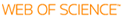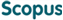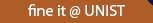### BROWSE

#### Related ResearcherSeo, Byoung Ki
Research Interests
• Financial Engineering, Finanicial Mathematics, Market Microstructure

Recurrence speed of multiples of an irrational number

Cited 8 times inCited 8 times inTitle
Recurrence speed of multiples of an irrational number
Other Titles
Recurrence speed of multiples of an irrational number
Author
Issue Date
2001-09
Publisher
Citation
PROCEEDINGS OF THE JAPAN ACADEMY SERIES A-MATHEMATICAL SCIENCES, v.77, no.7, pp.134 - 137
Abstract
Let 0 < < 1 be irrational and T()x = x + theta mod 1 on (0, 1). Consider the partition Q(n) = {((i-1)/2(n), i/2(n)) : 1 less than or equal to i less than or equal to 2(n)} and let Q(n)(x) denote the interval in Q(n) containing x. Define two versions of the first return time: J(n)(x) = min{j greater than or equal to 1 : parallel tox - T(theta)(j)x parallel to = parallel toj . theta parallel to < 1/2(n)} where t parallel to = min(n is an element ofZ) vertical bart -n vertical bar, and K-n(x) = min{j greater than or equal to 1 : T-theta(j) x is an element of Q(n)(x)}. We show that log J(n)/n --> 1 and log K-n(x)/n --> 1 a.e. as n --> infinity for a.e. theta
URI
https://scholarworks.unist.ac.kr/handle/201301/12169
URL
http://projecteuclid.org/euclid.pja/1148393039#info
DOI
10.3792/pjaa.77.134
ISSN
0386-2194
Appears in Collections:
SBA_Journal Papers
Files in This Item:
There are no files associated with this item.can give you direct access to the published full text of this article. (UNISTARs only)